# PyTorch实现神经网络### 一个神经元的神经网络

PyTorch中神经网络相关的layer称为module，封装在torch.nn中，由于我们的模型是线性的，我们可以用nn.Linear这个module，此外由于我们的例子中只有一个feature，加上我的输出也是一个值，因此我们的神经网络实际上只有一个神经元，输入是一个tensor，输出也是一个tensor。

import torch
import torch.nn as nn

t_x = [35.7, 55.9, 58.2, 81.9, 56.3, 48.9, 33.9, 21.8, 48.4, 60.4, 68.4]
t_x = torch.tensor(t_x).unsqueeze(1) #convert t_x to [11x1]
t_xn = t_x*0.1

linear_model = nn.Linear(1,1)
output = linear_model(t_xn)
print(output)


print("weight: ",linear_model.weight) #tensor([[-0.1335]], requires_grad=True)


optimizer = optim.SGD(linear_model.parameters(), lr=1e-2)
def train_loop(epochs, learning_rate, loss_fn,x, y):
for epoch in range(1, epochs + 1):
t_p = linear_model(x)
loss = loss_fn(y, t_p)
loss.backward()
optimizer.step()
print(f'Epoch: {epoch}, Loss: {float(loss)}')


1. 待训练参数$\omega$和$b$保存在linear_model.parameters()
2. 由于params保存在了model中，因此PyTorch知道如何update这些参数，不再需要我们手动编写梯度下降的代码
3. loss函数使用系统自带的nn.MSELoss对应上一节的L2 loss函数

train_loop(3000, 1e-2, nn.MSELoss(),t_xn, t_y)
print("params:", list(linear_model.parameters()))


### 非线性模型

seq_model = nn.Sequential(
nn.Linear(1,13),
nn.Tanh(),
nn.Linear(13,1))

print(seq_model)
# Sequential(
#   (0): Linear(in_features=1, out_features=13, bias=True)
#   (1): Tanh()
#   (2): Linear(in_features=13, out_features=1, bias=True)
# )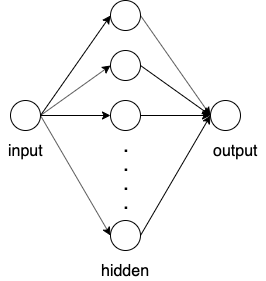for name,param in seq_model.named_parameters():
print(name, param.shape)

# 0.weight torch.Size([13, 1])
# 0.bias torch.Size()
# 2.weight torch.Size([1, 13])
# 2.bias torch.Size()


optimizer = optim.SGD(seq_model.parameters(), lr=1e-3)
def train_loop(epochs, learning_rate, loss_fn,x, y):
for epoch in range(1, epochs + 1):
t_p = seq_model(x)
loss = loss_fn(y, t_p)
loss.backward()
optimizer.step()
print(f'Epoch: {epoch}, Loss: {float(loss)}')

train_loop(5000, 1e-3, nn.MSELoss(),t_xn, t_y)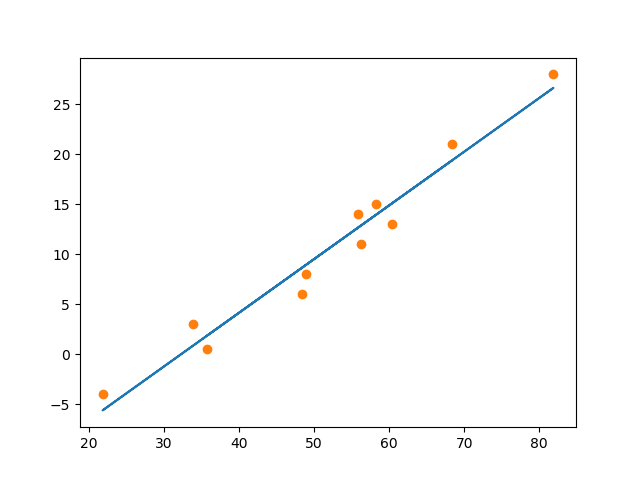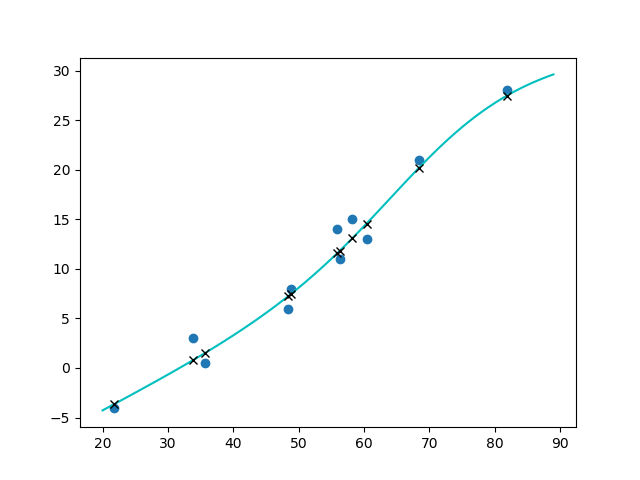### Fashion MNIST

from torchvision import datasets, transforms
# Define a transform to normalize the data
transform = transforms.Compose([transforms.ToTensor(),
transforms.Normalize((0.5,), (0.5,))])



print(len(trainloader)) #938
print(images.shape) #torch.Size([64, 1, 28, 28])
print(labels.shape) #torch.Size()


FC (784,256)
ReLU()
FC (256,128)
ReLU()
FC (128,64)
ReLU()
FC (64,10)
Softmax()


This criterion combines nn.LogSoftmax() and nn.NLLLoss() in one single class. The input is expected to contain scores for each class.

class Classifier(nn.Module):
def __init__(self):
super().__init__()
self.fc1 = nn.Linear(784, 256)
self.fc2 = nn.Linear(256, 128)
self.fc3 = nn.Linear(128, 64)
self.fc4 = nn.Linear(64, 10)
# Dropout module with 0.2 drop probability
self.dropout = nn.Dropout(p=0.2)

def forward(self, x):
x = x.view(x.shape, -1) #convert the input tensor to [64,784]
x = self.dropout(F.relu(self.fc1(x)))
x = self.dropout(F.relu(self.fc2(x)))
x = self.dropout(F.relu(self.fc3(x)))
x = F.log_softmax(self.fc4(x), dim=1)

return x

model = Classifier()
output = model(images)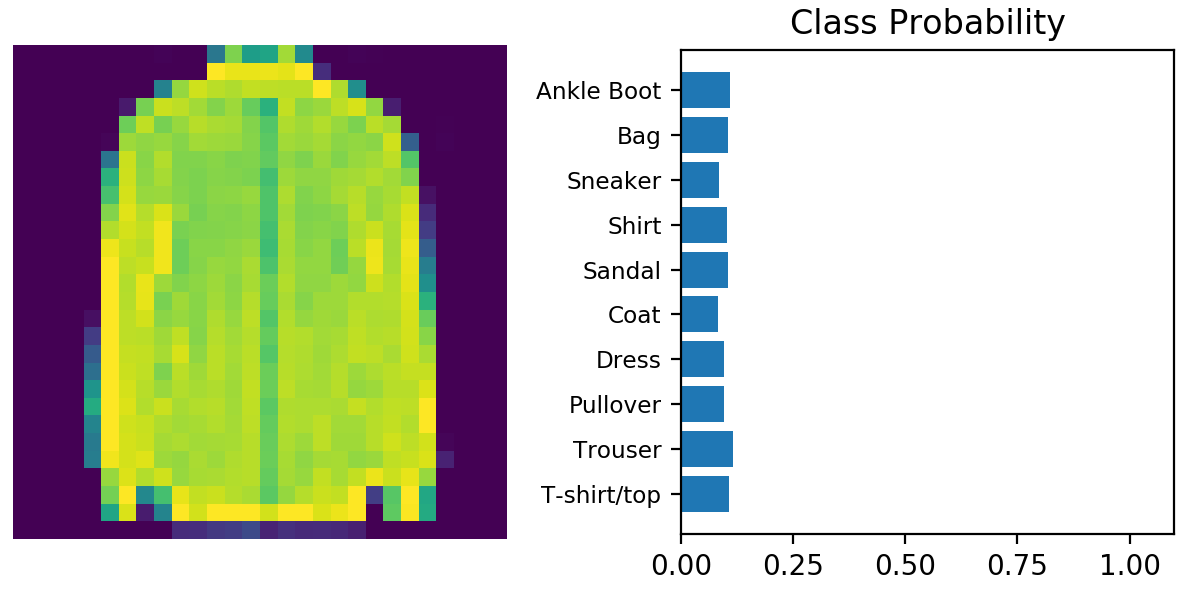loss_fn = nn.NLLLoss()
epochs = 5
for e in range(epochs):
running_loss = 0
outputs = model(images)
loss = loss_fn(outputs,labels)
loss.backward()
optimizer.step()
running_loss += loss.item()
else:
print(f"Traning loss: {running_loss}")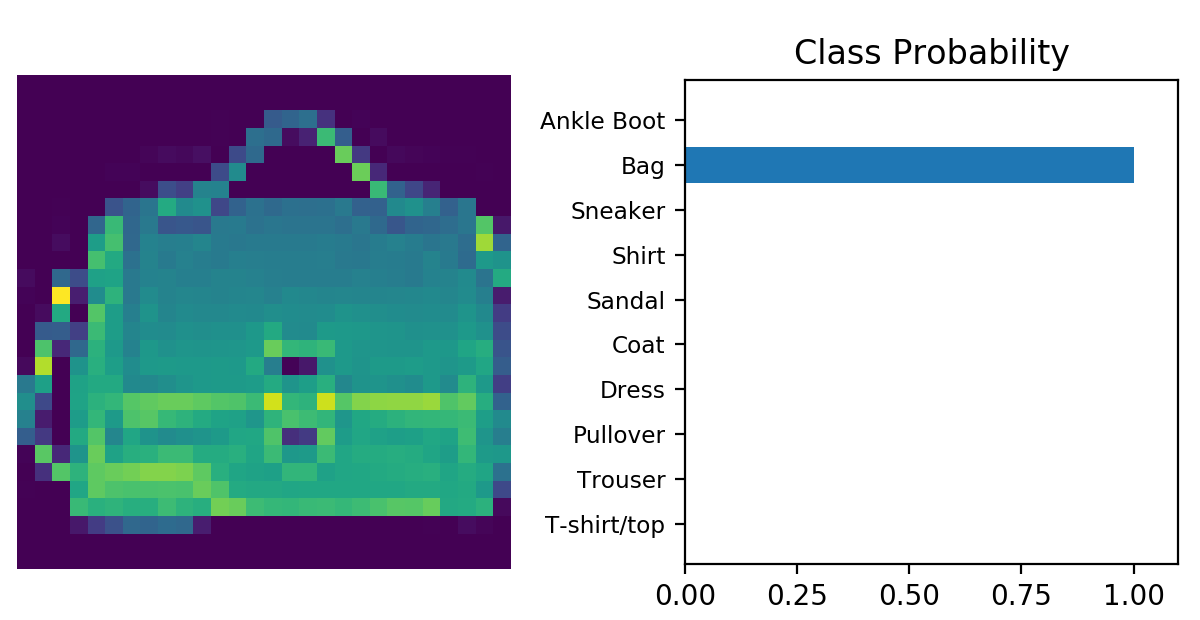### 解决过拟合问题

images, labels = next(iter(testloader))
output = torch.exp(model(images)) #convert the output tensor to [0,1]
top64 = output.topk(1,dim=1) #[64,1]
labels = labels.view(64,-1) #convert labels to [64.1]


equals = top64 == labels
accuracy = torch.mean(equals.type(torch.FloatTensor))


test_loss = 0
accuracy = 0
log_ps = model(images)
test_loss += loss_fn(log_ps, labels) #计算test_loss
ps = torch.exp(log_ps)
top_p, top_class = ps.topk(1, dim=1)
equals = top_class == labels.view(top_class.shape,-1)
accuracy += torch.mean(equals.type(torch.FloatTensor)) #计算accuracy
print("Epoch: {}/{}.. ".format(e+1, epochs),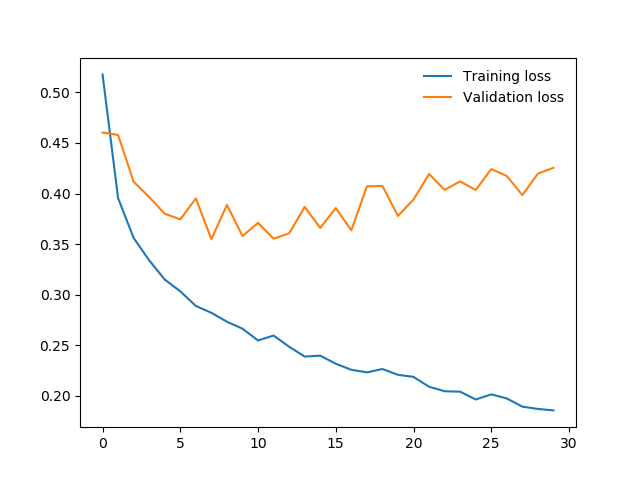class Classifier(nn.Module):
def __init__(self):
super().__init__()
self.fc1 = nn.Linear(784, 256)
self.fc2 = nn.Linear(256, 128)
self.fc3 = nn.Linear(128, 64)
self.fc4 = nn.Linear(64, 10)
# Dropout module with 0.2 drop probability
self.dropout = nn.Dropout(p=0.2)

def forward(self, x):
x = x.view(x.shape, -1)
x = self.dropout(F.relu(self.fc1(x)))
x = self.dropout(F.relu(self.fc2(x)))
x = self.dropout(F.relu(self.fc3(x)))
x = F.log_softmax(self.fc4(x), dim=1)

return x


with torch.no_grad():
model.eval() #disable dropout
...
#validation code
...
model.train()#enable dropout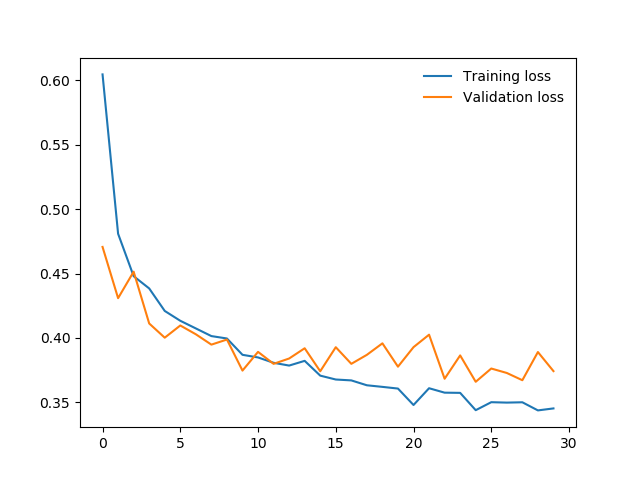### 小结

1. 准备数据，并可视化。这一步可以使用PyTorch的datasetstorch.utils.data.DataLoader
2. 对数据做预处理，这一步可以使用PyTorch的transforms
3. 设计model
4. 选取Optimizer和loss函数，训练
5. 观察training error和validation error，并在validation error区域稳定时停止training
6. 用测试集测试我们的model